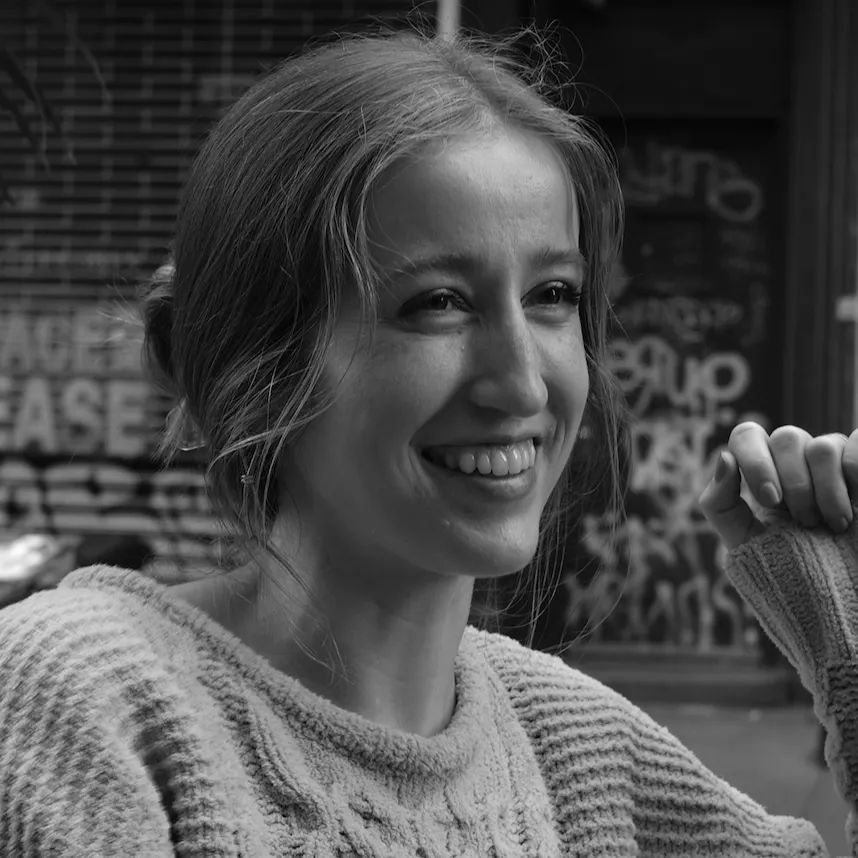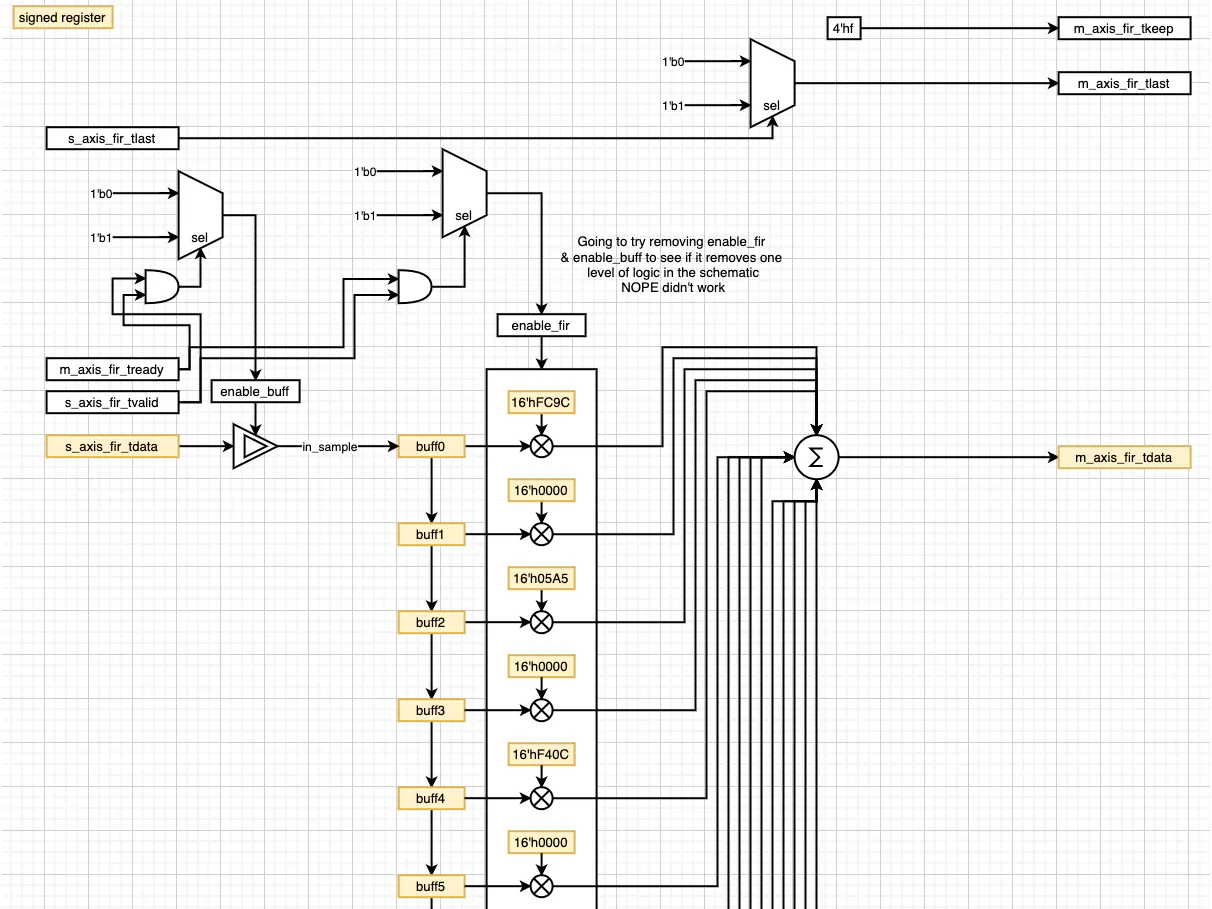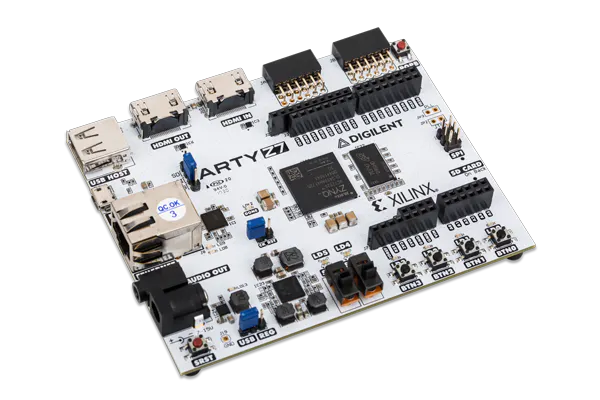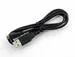# DSP for FPGA: Simple FIR Filter in Verilog

This project walks through how to implement a simple FIR filter with pre-generated coefficients in Verilog.

BeginnerFull instructions provided2 hours18,976## Things used in this project

### Hardware componentsDigilent Arty Z7-20
×1USB-A to Micro-USB Cable
×1

### Software apps and online services## Code

### FIR.v

Verilog
````timescale 1ns / 1ps

module FIR(
input clk,
input reset,
input signed [15:0] s_axis_fir_tdata,
input [3:0] s_axis_fir_tkeep,
input s_axis_fir_tlast,
input s_axis_fir_tvalid,
output reg m_axis_fir_tvalid,
output reg m_axis_fir_tlast,
output reg [3:0] m_axis_fir_tkeep,
output reg signed [31:0] m_axis_fir_tdata
);

always @ (posedge clk)
begin
m_axis_fir_tkeep <= 4'hf;
end

always @ (posedge clk)
begin
if (s_axis_fir_tlast == 1'b1)
begin
m_axis_fir_tlast <= 1'b1;
end
else
begin
m_axis_fir_tlast <= 1'b0;
end
end

// 15-tap FIR
reg enable_fir, enable_buff;
reg [3:0] buff_cnt;
reg signed [15:0] in_sample;
reg signed [15:0] buff0, buff1, buff2, buff3, buff4, buff5, buff6, buff7, buff8, buff9, buff10, buff11, buff12, buff13, buff14;
wire signed [15:0] tap0, tap1, tap2, tap3, tap4, tap5, tap6, tap7, tap8, tap9, tap10, tap11, tap12, tap13, tap14;
reg signed [31:0] acc0, acc1, acc2, acc3, acc4, acc5, acc6, acc7, acc8, acc9, acc10, acc11, acc12, acc13, acc14;

/* Taps for LPF running @ 1MSps with a cutoff freq of 400kHz*/
assign tap0 = 16'hFC9C;  // twos(-0.0265 * 32768) = 0xFC9C
assign tap1 = 16'h0000;  // 0
assign tap2 = 16'h05A5;  // 0.0441 * 32768 = 1445.0688 = 1445 = 0x05A5
assign tap3 = 16'h0000;  // 0
assign tap4 = 16'hF40C;  // twos(-0.0934 * 32768) = 0xF40C
assign tap5 = 16'h0000;  // 0
assign tap6 = 16'h282D;  // 0.3139 * 32768 = 10285.8752 = 10285 = 0x282D
assign tap7 = 16'h4000;  // 0.5000 * 32768 = 16384 = 0x4000
assign tap8 = 16'h282D;  // 0.3139 * 32768 = 10285.8752 = 10285 = 0x282D
assign tap9 = 16'h0000;  // 0
assign tap10 = 16'hF40C; // twos(-0.0934 * 32768) = 0xF40C
assign tap11 = 16'h0000; // 0
assign tap12 = 16'h05A5; // 0.0441 * 32768 = 1445.0688 = 1445 = 0x05A5
assign tap13 = 16'h0000; // 0
assign tap14 = 16'hFC9C; // twos(-0.0265 * 32768) = 0xFC9C

/* This loop sets the tvalid flag on the output of the FIR high once
* the circular buffer has been filled with input samples for the
* first time after a reset condition. */
always @ (posedge clk or negedge reset)
begin
if (reset == 1'b0) //if (reset == 1'b0 || tvalid_in == 1'b0)
begin
buff_cnt <= 4'd0;
enable_fir <= 1'b0;
in_sample <= 8'd0;
end
else if (m_axis_fir_tready == 1'b0 || s_axis_fir_tvalid == 1'b0)
begin
enable_fir <= 1'b0;
buff_cnt <= 4'd15;
in_sample <= in_sample;
end
else if (buff_cnt == 4'd15)
begin
buff_cnt <= 4'd0;
enable_fir <= 1'b1;
in_sample <= s_axis_fir_tdata;
end
else
begin
buff_cnt <= buff_cnt + 1;
in_sample <= s_axis_fir_tdata;
end
end

always @ (posedge clk)
begin
if(reset == 1'b0 || m_axis_fir_tready == 1'b0 || s_axis_fir_tvalid == 1'b0)
begin
m_axis_fir_tvalid <= 1'b0;
enable_buff <= 1'b0;
end
else
begin
m_axis_fir_tvalid <= 1'b1;
enable_buff <= 1'b1;
end
end

/* Circular buffer bring in a serial input sample stream that
* creates an array of 15 input samples for the 15 taps of the filter. */
always @ (posedge clk)
begin
if(enable_buff == 1'b1)
begin
buff0 <= in_sample;
buff1 <= buff0;
buff2 <= buff1;
buff3 <= buff2;
buff4 <= buff3;
buff5 <= buff4;
buff6 <= buff5;
buff7 <= buff6;
buff8 <= buff7;
buff9 <= buff8;
buff10 <= buff9;
buff11 <= buff10;
buff12 <= buff11;
buff13 <= buff12;
buff14 <= buff13;
end
else
begin
buff0 <= buff0;
buff1 <= buff1;
buff2 <= buff2;
buff3 <= buff3;
buff4 <= buff4;
buff5 <= buff5;
buff6 <= buff6;
buff7 <= buff7;
buff8 <= buff8;
buff9 <= buff9;
buff10 <= buff10;
buff11 <= buff11;
buff12 <= buff12;
buff13 <= buff13;
buff14 <= buff14;
end
end

/* Multiply stage of FIR */
always @ (posedge clk)
begin
if (enable_fir == 1'b1)
begin
acc0 <= tap0 * buff0;
acc1 <= tap1 * buff1;
acc2 <= tap2 * buff2;
acc3 <= tap3 * buff3;
acc4 <= tap4 * buff4;
acc5 <= tap5 * buff5;
acc6 <= tap6 * buff6;
acc7 <= tap7 * buff7;
acc8 <= tap8 * buff8;
acc9 <= tap9 * buff9;
acc10 <= tap10 * buff10;
acc11 <= tap11 * buff11;
acc12 <= tap12 * buff12;
acc13 <= tap13 * buff13;
acc14 <= tap14 * buff14;
end
end

/* Accumulate stage of FIR */
always @ (posedge clk)
begin
if (enable_fir == 1'b1)
begin
m_axis_fir_tdata <= acc0 + acc1 + acc2 + acc3 + acc4 + acc5 + acc6 + acc7 + acc8 + acc9 + acc10 + acc11 + acc12 + acc13 + acc14;
end
end

endmodule
```

### tb_FIR.v

Verilog
````timescale 1ns / 1ps

module tb_FIR;

reg signed [15:0] s_axis_fir_tdata;
wire m_axis_fir_tvalid;
wire [3:0] m_axis_fir_tkeep;
wire [31:0] m_axis_fir_tdata;

/*
* 100Mhz (10ns) clock
*/
always begin
clk = 1; #5;
clk = 0; #5;
end

always begin
reset = 1; #20;
reset = 0; #50;
reset = 1; #1000000;
end

always begin
s_axis_fir_tvalid = 0; #100;
s_axis_fir_tvalid = 1; #1000;
s_axis_fir_tvalid = 0; #50;
s_axis_fir_tvalid = 1; #998920;
end

always begin
end

/* Instantiate FIR module to test. */
FIR FIR_i(
.clk(clk),
.reset(reset),
.s_axis_fir_tdata(s_axis_fir_tdata),
.s_axis_fir_tkeep(s_axis_fir_tkeep),
.s_axis_fir_tlast(s_axis_fir_tlast),
.s_axis_fir_tvalid(s_axis_fir_tvalid),
.m_axis_fir_tvalid(m_axis_fir_tvalid),
.m_axis_fir_tlast(m_axis_fir_tlast),
.m_axis_fir_tkeep(m_axis_fir_tkeep),
.m_axis_fir_tdata(m_axis_fir_tdata));

reg [4:0] state_reg;
reg [3:0] cntr;

parameter wvfm_period = 4'd4;

parameter init               = 5'd0;
parameter sendSample0        = 5'd1;
parameter sendSample1        = 5'd2;
parameter sendSample2        = 5'd3;
parameter sendSample3        = 5'd4;
parameter sendSample4        = 5'd5;
parameter sendSample5        = 5'd6;
parameter sendSample6        = 5'd7;
parameter sendSample7        = 5'd8;

/* This state machine generates a 200kHz sinusoid. */
always @ (posedge clk or posedge reset)
begin
if (reset == 1'b0)
begin
cntr <= 4'd0;
s_axis_fir_tdata <= 16'd0;
state_reg <= init;
end
else
begin
case (state_reg)
init : //0
begin
cntr <= 4'd0;
s_axis_fir_tdata <= 16'h0000;
state_reg <= sendSample0;
end

sendSample0 : //1
begin
s_axis_fir_tdata <= 16'h0000;

if (cntr == wvfm_period)
begin
cntr <= 4'd0;
state_reg <= sendSample1;
end
else
begin
cntr <= cntr + 1;
state_reg <= sendSample0;
end
end

sendSample1 : //2
begin
s_axis_fir_tdata <= 16'h5A7E;

if (cntr == wvfm_period)
begin
cntr <= 4'd0;
state_reg <= sendSample2;
end
else
begin
cntr <= cntr + 1;
state_reg <= sendSample1;
end
end

sendSample2 : //3
begin
s_axis_fir_tdata <= 16'h7FFF;

if (cntr == wvfm_period)
begin
cntr <= 4'd0;
state_reg <= sendSample3;
end
else
begin
cntr <= cntr + 1;
state_reg <= sendSample2;
end
end

sendSample3 : //4
begin
s_axis_fir_tdata <= 16'h5A7E;

if (cntr == wvfm_period)
begin
cntr <= 4'd0;
state_reg <= sendSample4;
end
else
begin
cntr <= cntr + 1;
state_reg <= sendSample3;
end
end

sendSample4 : //5
begin
s_axis_fir_tdata <= 16'h0000;

if (cntr == wvfm_period)
begin
cntr <= 4'd0;
state_reg <= sendSample5;
end
else
begin
cntr <= cntr + 1;
state_reg <= sendSample4;
end
end

sendSample5 : //6
begin
s_axis_fir_tdata <= 16'hA582;

if (cntr == wvfm_period)
begin
cntr <= 4'd0;
state_reg <= sendSample6;
end
else
begin
cntr <= cntr + 1;
state_reg <= sendSample5;
end
end

sendSample6 : //6
begin
s_axis_fir_tdata <= 16'h8000;

if (cntr == wvfm_period)
begin
cntr <= 4'd0;
state_reg <= sendSample7;
end
else
begin
cntr <= cntr + 1;
state_reg <= sendSample6;
end
end

sendSample7 : //6
begin
s_axis_fir_tdata <= 16'hA582;

if (cntr == wvfm_period)
begin
cntr <= 4'd0;
state_reg <= sendSample0;
end
else
begin
cntr <= cntr + 1;
state_reg <= sendSample7;
end
end

endcase
end
end

endmodule
```

## Credits

### Whitney Knitter

78 projects • 890 followers
Working as a full-time SDR/FPGA engineer, but making time for the fun projects at home.